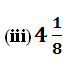## EX 1.3 QUESTION 1.

Write the following in decimal form and say what kind of decimal expansion each has :

(i) 36/100

(ii)1/11(iv) 3/13

(v) 2/11

(vi) 329/400

Solution:## EX 1.3 QUESTION 2.

You know that 1/7 = 0.142857. Can you predict what the decimal expansions of 2/7, 3/7, 4/7, 5/7, 6/7 are, without actually doing the long division? If so, how?

[Hint: Study the remainders while finding the value of 1/7 carefully.]

Solution:## EX 1.3 QUESTION 3.

3. Express the following in the form p/q, where p and q are integers and q 0.

(i) 0.$\bar { 6 }$
(ii) 0.4$\bar { 7 }$
(iii) 0.$\overline { 001 }$
Solution:

(i) 0.$\bar { 6 }$

Let x = 0.$\bar { 6 }$

x = 0.6666……….. (i)

multiplying equation (i) by 10 on both sides,

10x = 6.666…

10x = 6 + x    [From equation (I)]

9x = 6

x = 6/9

x = 2/3

(ii) 0.4$\bar { 7 }$

Let x = 0.4$\bar { 7 }$

x = 0.47777………….. (i)

multiplying equation (i) by 10 on both sides,

10x = 4.7777 ………….. (ii)

multipmultiplying equation (ii) by 100 on both sides,

100x = 47.7777…

100x = 43 + 47.7777

100x = 43 + 10x      [From equation (Ii)]

100x – 10 x = 43

90x = 43

x = 43/90

(iii) 0.$\overline { 001 }$

Let x = 0.$\overline { 001 }$

x = 0.001001001…………(i)

multiplying equation (i) by 1000 on both sides,

1000x =1.001001001……

⇒ 1000x =  1 + 0.001001001…..

⇒ 1000x =1 + x                [from equation (i)]

⇒ 1000x = 1

⇒ 999x = 1

x = 1/ 999

## EX 1.3 QUESTION 4.

Express 0.99999…. in the form p/q. Are you surprised by your answer? With your teacher and classmates discuss why the answer makes sense.

Solution:

Let x= 0.99999………..(i)

multiplying equation (i) by 10 on both sides,

10x = 9.99999

⇒ 10x = 9+ 0.99999

⇒ 10x = 9+ x        [from equation (i)]

⇒ 10x = 9

⇒ 9x = 9

x = 9/9 = 1

x = 1

The difference between 1 and 0.999999 is 0.000001 which is very close.

Hence, we can say that 0.99999 = 1.

## EX 1.3 QUESTION 5.

What can the maximum number of digits be in the repeating block of digits in the decimal expansion of 1/17? Perform the division to check your answer.

Solution:

1/171/17 =$\overline { 0588235294117647 }$

There are maximum16 digits in the repeating block of the decimal expansion of 1/17.

## EX 1.3 QUESTION 6.

Look at several examples of rational numbers in the form p/q (q ≠ 0), where p and q are integers with no common factors other than 1 and having terminating decimal representations (expansions). Can you guess what property q must satisfy?

Solution:

• 2/5 = 0.4
• 1/10 = 0.1
• 3/2 = 1.5
• 7/8 = 0.875

The denominator of all the rational numbers is in front of 2m x 5n , where m and n are integers.

## EX 1.3 QUESTION 7.

Write three numbers whose decimal expansions are non-terminating non-recurring.

Solution:

1. √3 = 1.732050807568
2. √5 = 2.23606797
3. √26 =5.099019513592

## EX 1.3 QUESTION 8.

8. Find three different irrational numbers between the rational numbers 5/7 and 9/11.

Solution:Three different irrational numbers between 0.$\overline { 714285 }$ and  0.$\overline { 81 }$ are

1. 0.73073007300073000073…
2. 0.75075007300075000075…
3. 0.76076007600076000076…

## EX 1.3 QUESTION 9.

Classify the following numbers as rational or irrational according to their type:

(i) √23

(ii)√225

(iii) 0.3796

(iv) 7.478478…..

(v) 1.101001000100001

Solution:

(i) √23

√23 = 4.79583152331…

Irrational number.

(ii)√225

√225 = 15 = 15/1

Rational number.

(iii) 0.3796

Rational number.

(iv) 7.478478…..

Rational number.

(v) 1.101001000100001

Irrational number.

Categories: class 9NCERT NOTES

error: Content is protected !!